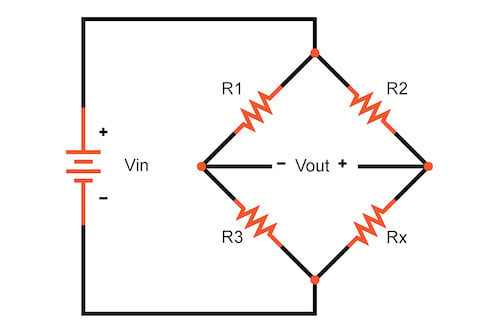# Wheatstone Bridge Calculator

## This calculator helps determine the resistance of a variable resistor within a Wheatstone bridge circuit or the differential voltage created across the bridge terminals.

### You must input everything except for either Rx or Vout

This calculator can be used to either design a Wheatstone bridge or to interpret the output of a sensor within a Wheatstone bridge. As shown in Figure 1, the Wheatstone bridge is a simple circuit consisting of two sets of series resistors connected in parallel##### Figure 1. A Wheatstone bridge circuit

The Wheatstone bridge configuration allows for high accuracy measurements of unknown resistance.

### Balancing a Wheatstone Bridge Circuit

This type of circuit is balanced when:

$$V_{out} = 0$$

To achieve this balance, the resistance ratios in the circuit must be balanced:

$$\frac{R_1}{R_3} = \frac{R_2}{R_x}$$

Even a small change in resistance (Rx) causes the bridge to become unbalanced. These changes result in a non-zero voltage at Vout with the polarity indicating if Rx is smaller or larger than the balanced value. Utilizing a differential amplifier across the Vout terminals to extract the differential signal while rejecting common-mode noise makes precise detection of very small changes possible.

This calculator with either solve for Vout in the figure above given the Rx resistance or solve for the Rx resistance given Vout.

### Wheatstone Bridge Equation—Using KVL and KCL

To solve for output voltage, Vout, given the Rx resistance, we use Kirchoff's Voltage Law (KVL) and Kirchoff's Current Law (KCL) to arrive at the following equation:

$$V_{out} = V_{in} \centerdot (\frac{R_x}{R_3+R_x} - \frac{R_2}{R_1+R_2})$$

Where:

• Vout = differential voltage across the Wheatstone bridge, in volts
• Vin = input voltage to the Wheatstone bridge, in volts
• Rn = resistor values, expressed in ohms

Similarly, to solve for Rx given the output voltage Vout, we'll use KVL and KCL to arrive at the following equation:

$$R_{x} = \frac{R_2 R_3 + R_3 \centerdot(R_1+R_2) \centerdot \frac{V_{out}}{V_{in}}}{R_1 - (R_1+R_2) \centerdot \frac{V_{out}}{V_{in}}}$$

### Further Reading on the Wheatstone Bridge